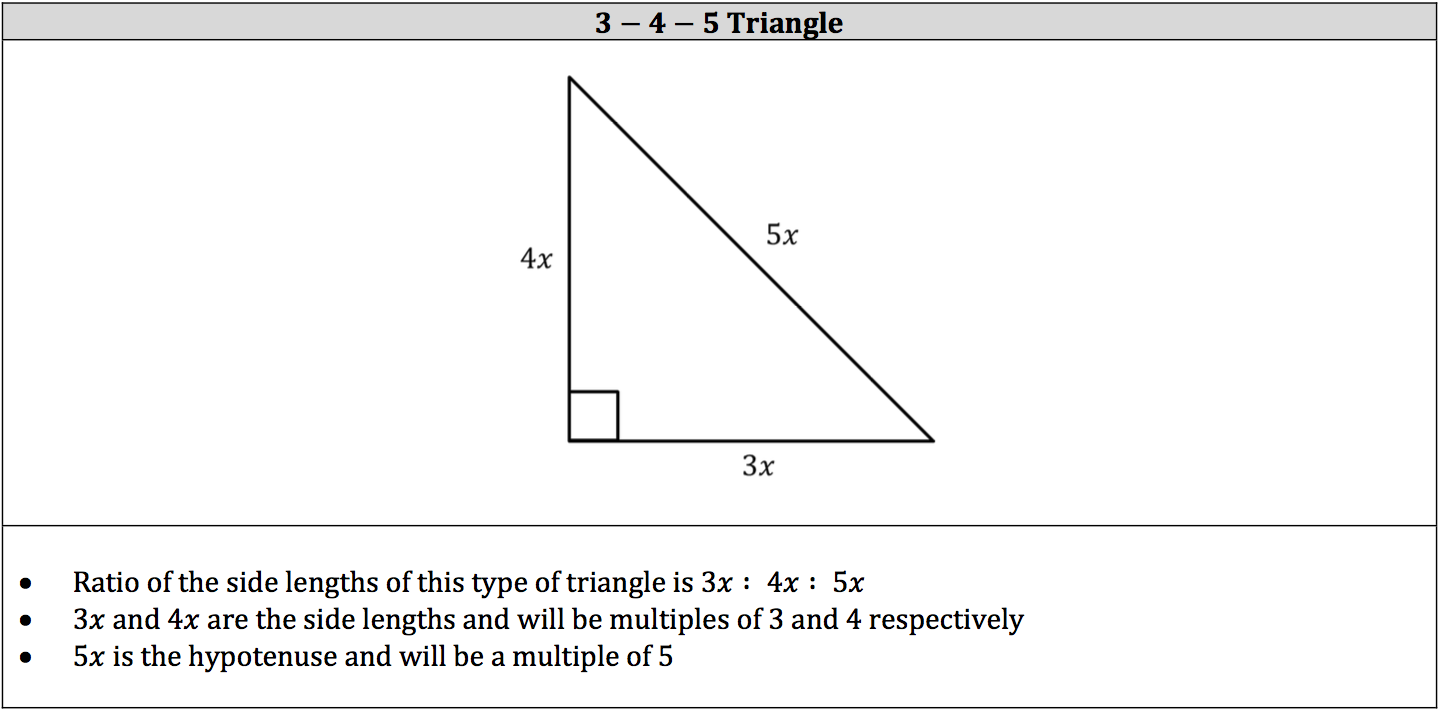Triangles are those handy‐dandy three‐sided polygons with which mathematicians have done so much work, particularly the right triangle.

The most important thing to remember about all triangles is that the sum of their interior angles is 180°. Again, the sum of the interior angles of any triangle is 180°. Once more, the sum of the interior angles of any triangle is 180°!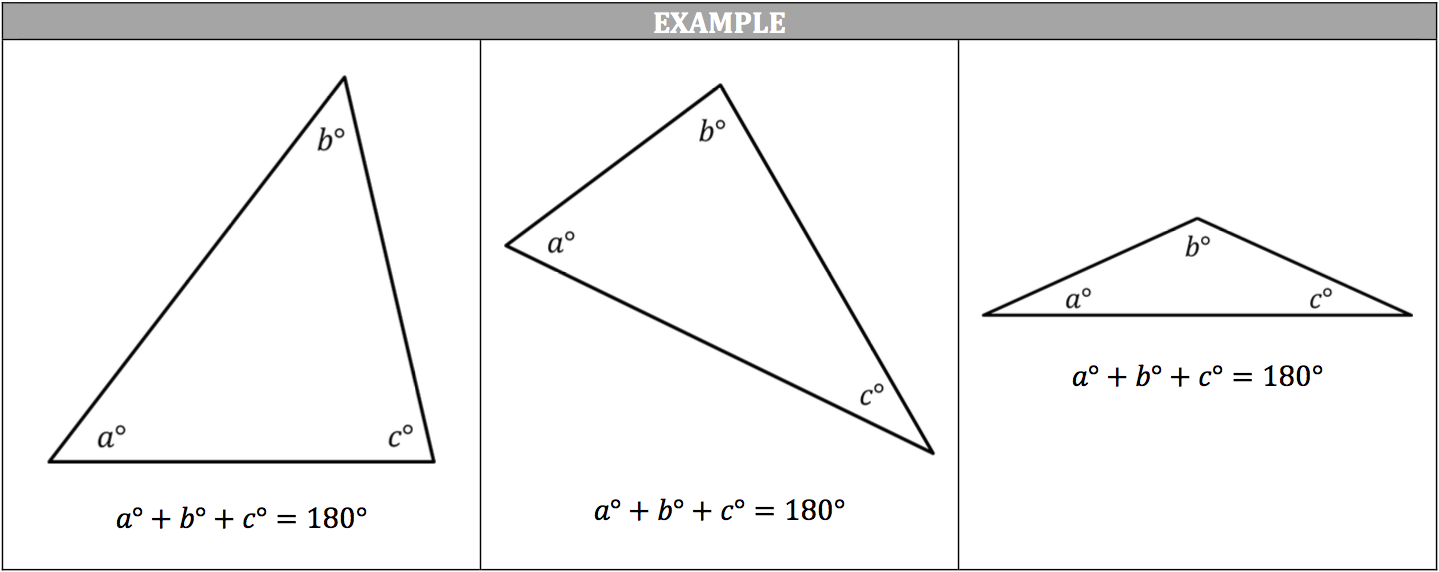## Triangle Inequality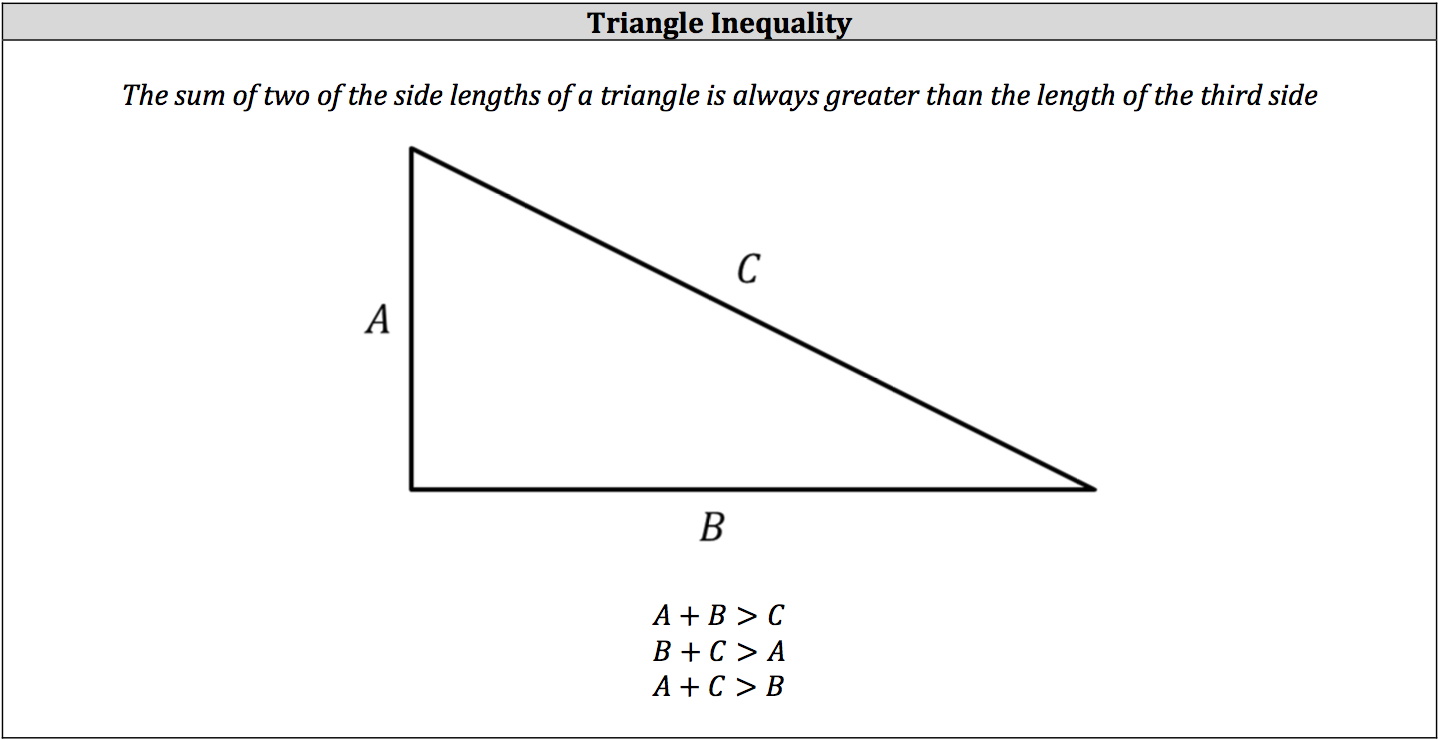## Angles and Their Side Lengths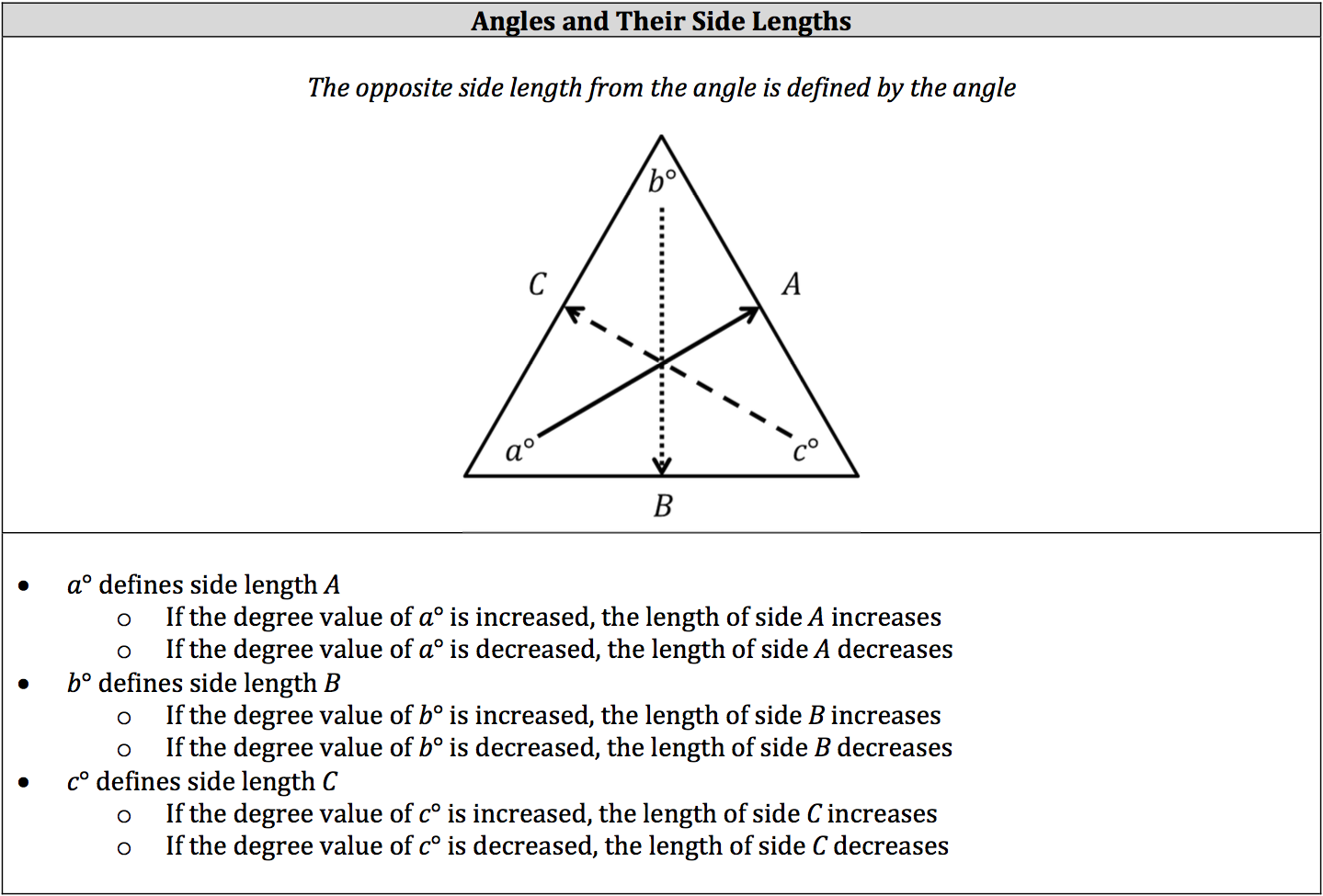## Equilateral Triangle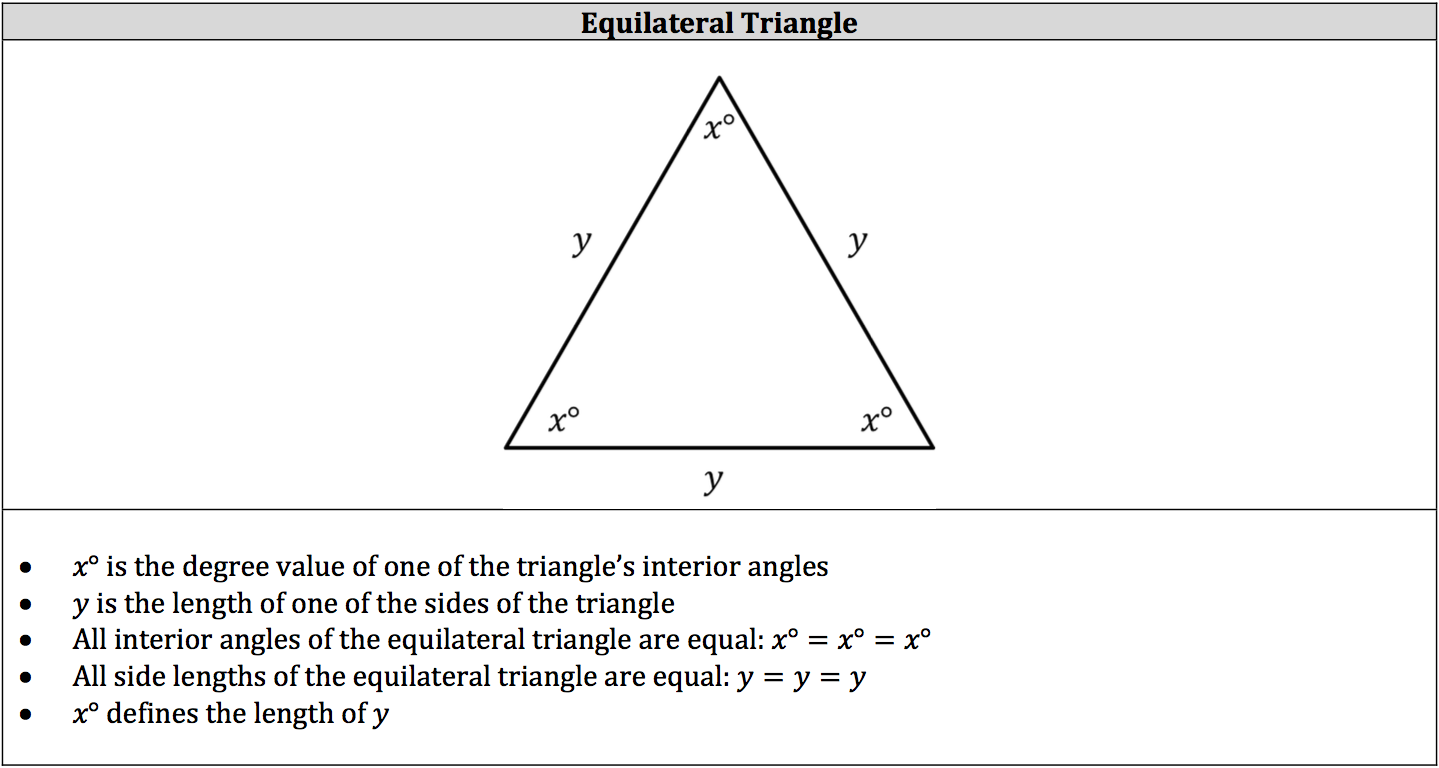## Isosceles Triangle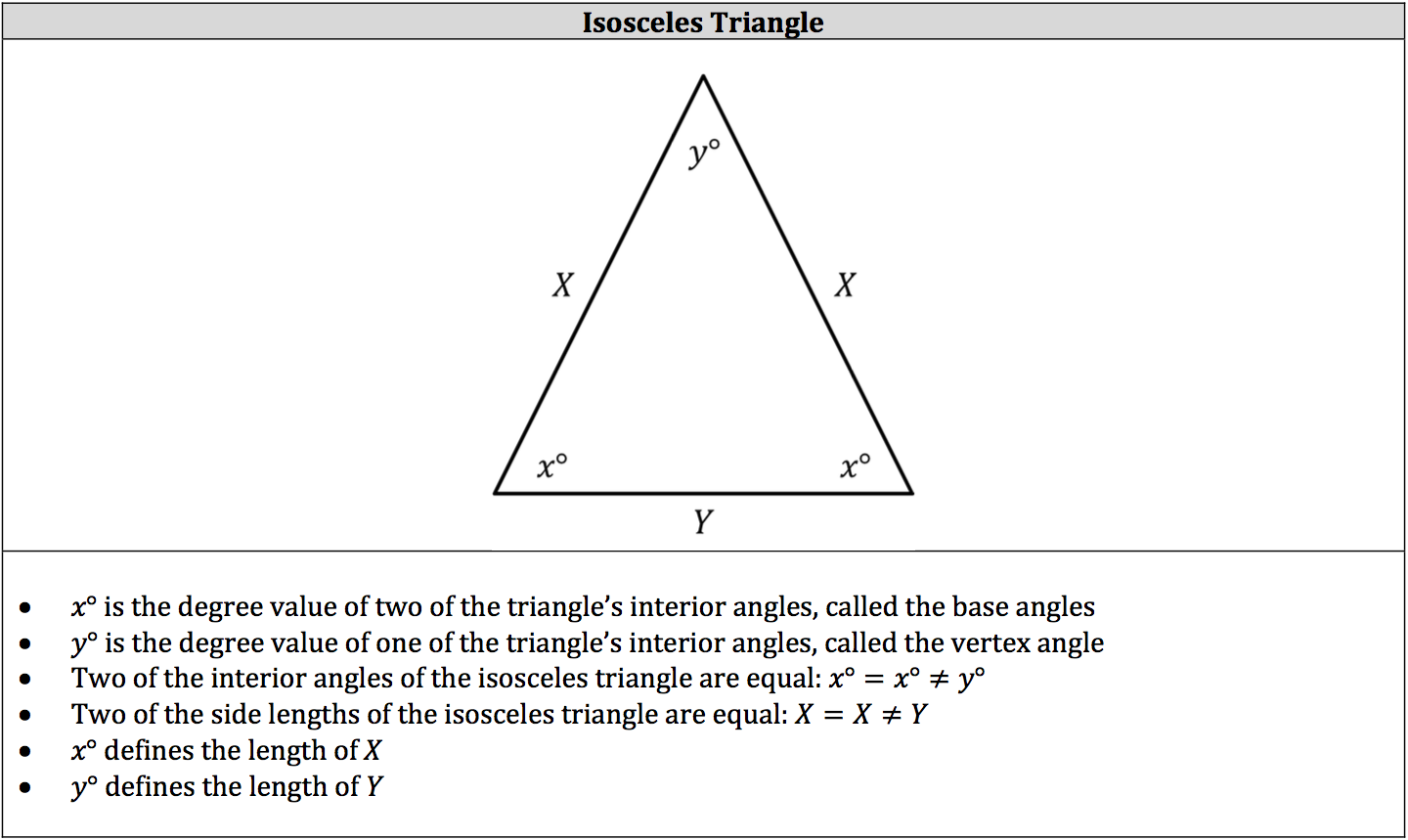## Scalene Triangle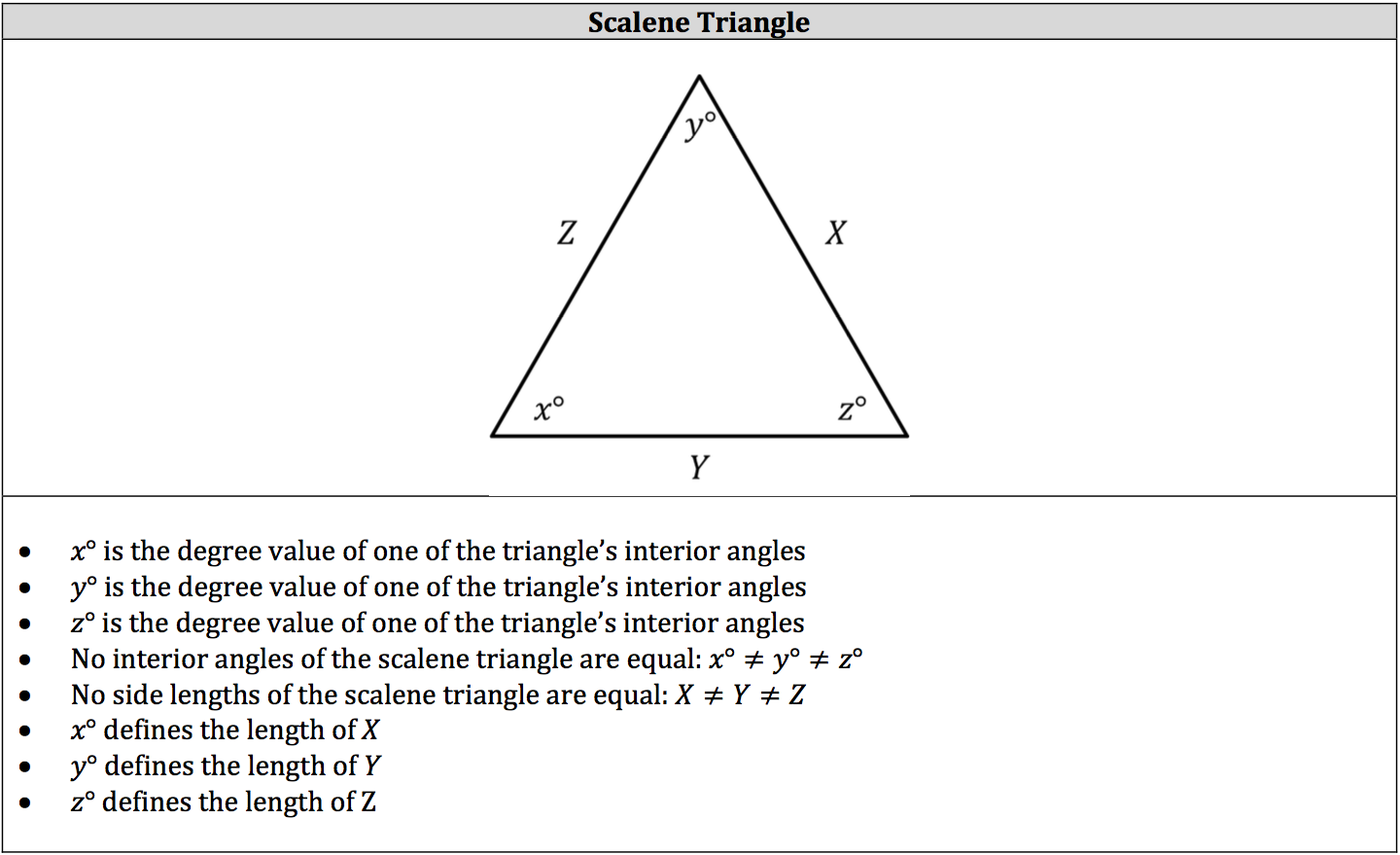## Right Triangle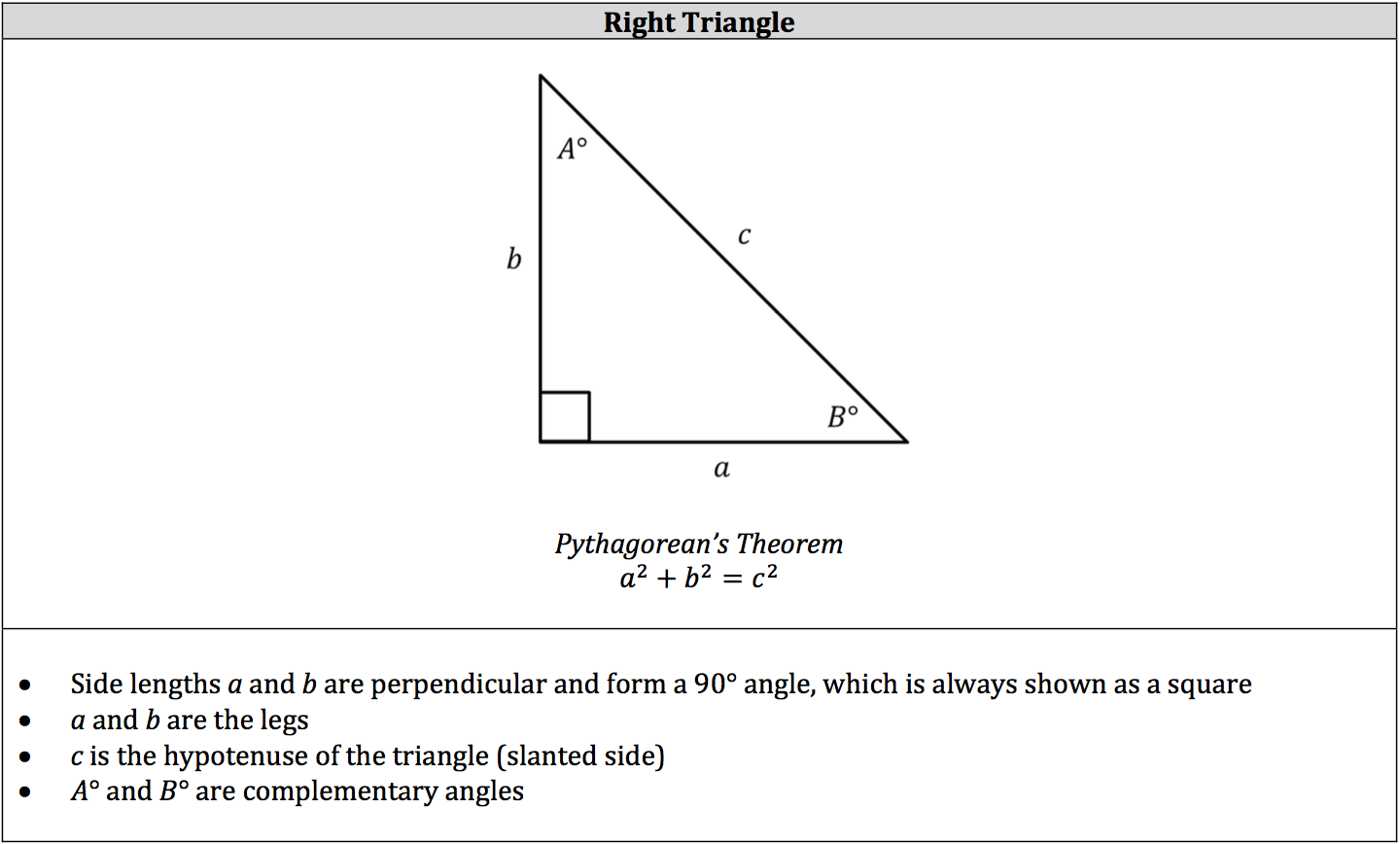### 45° – 45° – 90° Triangle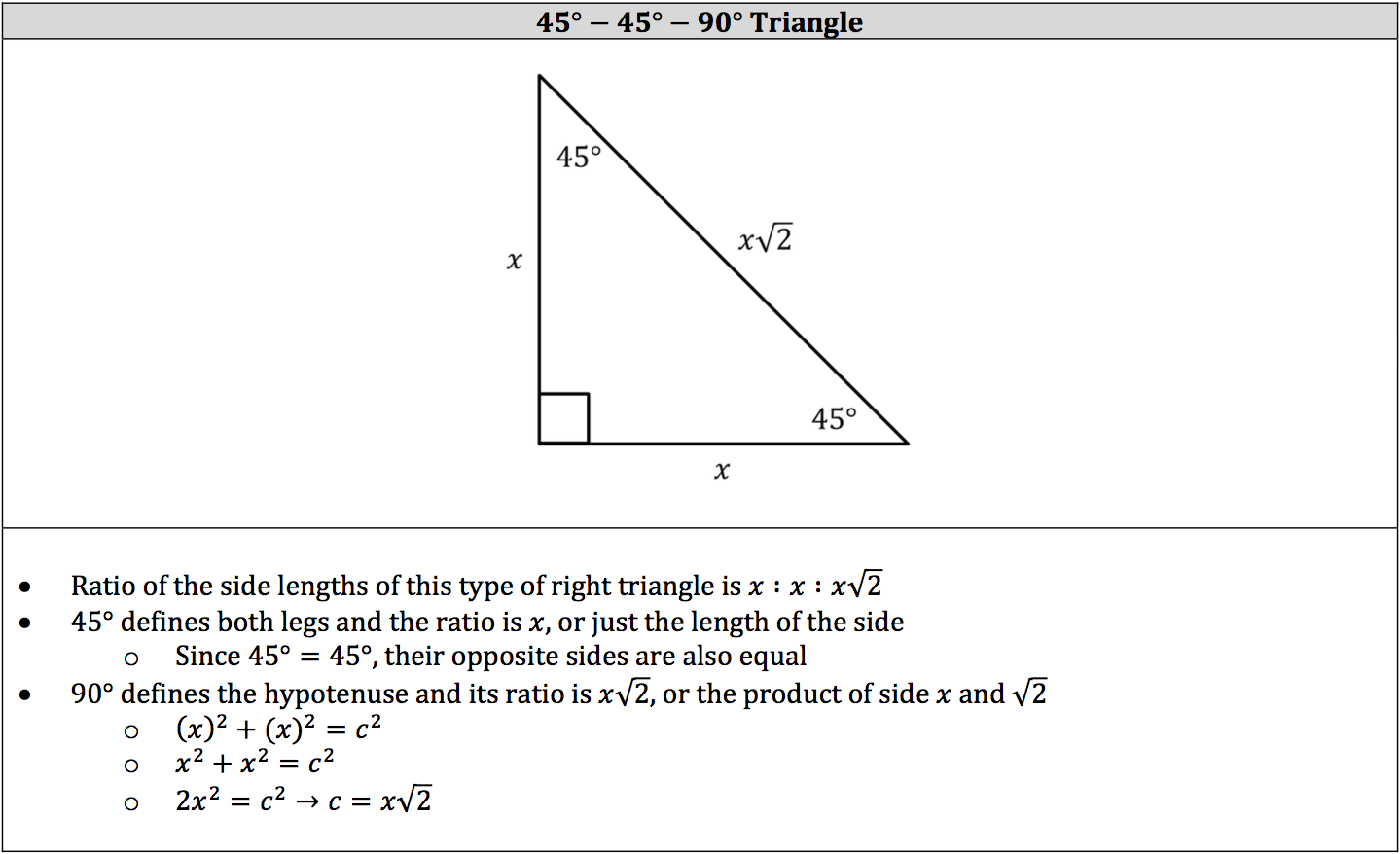### 3 – 4 – 5 Triangle